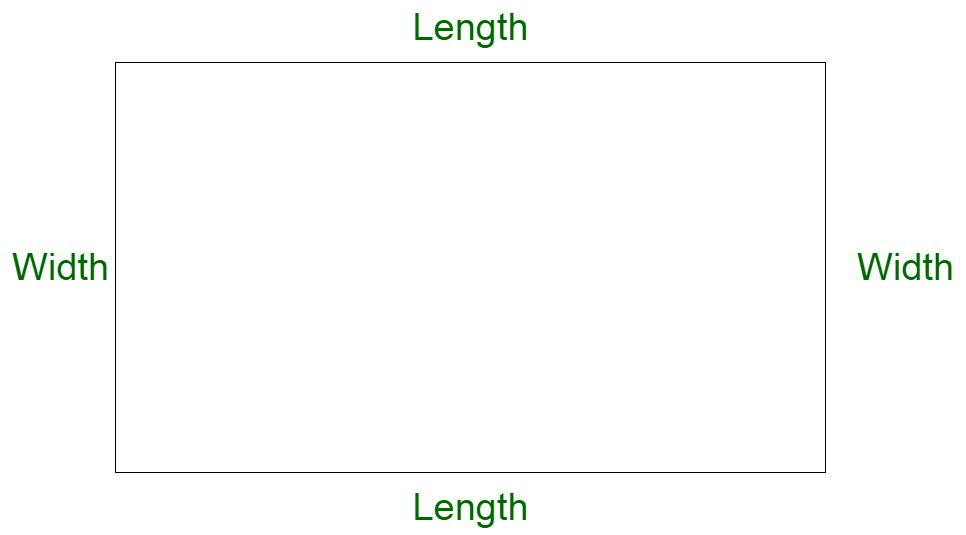# What is the length of the Rectangle whose Perimeter is 24 cm and Width is 3 cm?

• Last Updated : 20 Nov, 2021

Rectangle is a closed two-dimensional figure composed of four sides and four vertices. All angles of the rectangle are 90 degrees. A rectangle with all sides equal is equivalent to a square. A rectangle is composed of two pairs of parallel sides, length, and width respectively.Perimeter of Rectangle

The perimeter of a rectangle is the length of the outer boundary of a rectangle. It is also calculated by the summation of the total measure of both the lengths and breadths of the rectangle.

Perimeter of Rectangle Formula

Let us assume a rectangle of perimeter P, whose length and width are ‘l’ and ‘w’ respectively is 2(l + w).

Perimeter of a Rectangle Formula = 2 (Length + Width) units

### What is the Length of the Rectangle whose Perimeter is 24 cm and Width is 3 cm?

Solution:

We have,

Length, l of the rectangle =3 cm

Perimeter of the rectangle, P = 24 cm

We have,

Perimeter of a Rectangle Formula = 2 (Length + Width) units

Let us assume w to be the width of rectangle.

Now,

P = 2(l + w) cm

Substituting the values, we get,

P = 2(3 + w) cm

24 = (6 + 2w) cm

On solving, we get,

18 = 2w

w = 9 cm

Therefore, the width of rectangle is equivalent to 9 cm.

### Sample Questions

Question 1: Compute the general formula of the width of the rectangle in terms of perimeter P and length l.

Solution:

Let us assume w to be the width of the rectangle.

We have,

P = 2(l + w)

P/2 = ( l + w)

On rearranging, we get,

w =Question 2: Compute the perimeter of the rectangle where length and breadth are 2 and 3 cm respectively.

Solution:

We know,

P = 2(l + w)

P = 2 (2 + 3) cm

P = 2 (5) cm

= 10 cm

Question 3: Compute the length of the rectangle where the perimeter is 20 cm and all sides are equivalent.

Solution:

Let us assume s to be the side of the rectangle.

Now,

P = 2(l + w)

We know,

l = w = s

Therefore,

P = 2(2s)

P = 4s

Substituting, P = 20 cm

s = 20/4 cm

= 5 cm

My Personal Notes arrow_drop_up